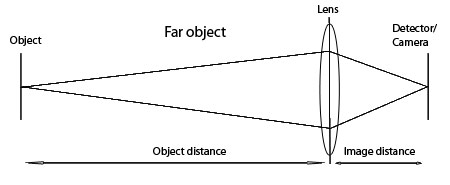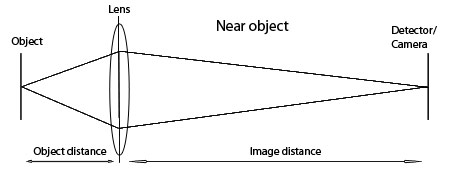# Basic ImageFormation

back

Several things come out of this equation that are useful to understand. As the object distance extends to infinity, 1/o tends toward zero. That means that the image distance equals the focal length. To focus at infinity, the center of the lens needs to be the focal length away from the detector. On the opposite end, as the image distance approaches infinity, 1/i approaches zero. The object distance equals the focal length in front of the lens. This means that the minimum working distance of a lens is the focal length in front of the center of the lens. A longer focal length lens will have a larger minimum working distance compared to a shorter focal length lens and will have more working distance at all magnification levels.

In practical terms, a traditional camera lens (not a newer internal focus lens) will be at its shortest length when it focuses on something in the distance - the image distance is minimized. Conversely when focusing on something close, the lens will have to lengthen, increasing the image distance.

Now that we have discussed the issue of focal length we can move on to basic image formation. There is a mathematical relationship between the distance from the object to the lens and from the lens to the image - i.e. object distance vs. image distance

1/f = 1/o + 1/i (where f = focal length, o = object distance, i = image distance)